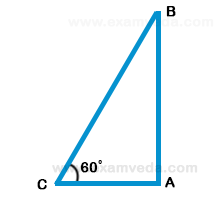# The angle of elevation of a ladder leaning against a wall is 60º and the foot of the ladder is 4.6 m away from the wall. The length of the ladder is:

A. 2.3 m

B. 4.6 m

C. 7.8 m

D. 9.2 m

### Solution(By Examveda Team)

Let AB be the wall and BC be the ladder.Then, ∠ACB = 60° = AC = 4.6m
$$\frac{{AC}}{{BC}} = \cos {60^ \circ } = \frac{1}{2}$$
⇒ BC = 2 × AC = 2 × 4.6 = 9.2m

This Question Belongs to Arithmetic Ability >> Height And Distance

1.